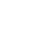###### Wealthy AffiliateRank 22459
274 followers Joined March 2016Hi, My name's Karen. I just moved across country to be near my elderly parents. I rented a house sight unseen on craigslist, packedPosts

2Questions

1Create Your Free Wealthy Affiliate Account Today!4-Steps to Success ClassMarket Research & Analysis ToolsMillionaire MentorshipI want to create & add a calculator in my website. tips?

I want to build a tool for users to calculate the size of the rainwater storage tank appropriate for them. I can incorporate some detail if they do things like plug in their z

This is untested by me but I got it from wiki how by searching for html calculator.

<html>
<title>HTML Calculator</title>
<body bgcolor= "#000000" text= "gold">
<form name="calculator" >
<input type="button" value="1">
<input type="button" value="2">
<input type="button" value="3">
<input type="button" value="+">

<input type="button" value="4">
<input type="button" value="5">
<input type="button" value="6">
<input type="button" value="-">

<input type="button" value="7">
<input type="button" value="8">
<input type="button" value="9">
<input type="button" value="*">
<input type="button" value="/">

<input type="button" value="0">
<input type="reset" value="Reset">
<input type="button" value="=">
Solution is <input type="textfield" name="ans" value="">
</form>

</body>
</html>I want to create & add a calculator in my website. tips?

I want to build a tool for users to calculate the size of the rainwater storage tank appropriate for them. I can incorporate some detail if they do things like plug in their z

This is untested by me but I got it from wiki how by searching for html calculator.

<html>
<title>HTML Calculator</title>
<body bgcolor= "#000000" text= "gold">
<form name="calculator" >
<input type="button" value="1">
<input type="button" value="2">
<input type="button" value="3">
<input type="button" value="+">

<input type="button" value="4">
<input type="button" value="5">
<input type="button" value="6">
<input type="button" value="-">

<input type="button" value="7">
<input type="button" value="8">
<input type="button" value="9">
<input type="button" value="*">
<input type="button" value="/">

<input type="button" value="0">
<input type="reset" value="Reset">
<input type="button" value="=">
Solution is <input type="textfield" name="ans" value="">
</form>

</body>
</html>Create Your Free Wealthy Affiliate Account Today!4-Steps to Success Class